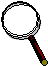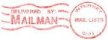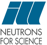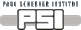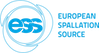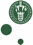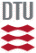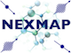McStas: Monochromator_pol Component

# The `Monochromator_pol` Component

Flat polarizaing monochromator crystal.

## Identification

• Author: Peter Christiansen
• Origin: RISOE
• Date: 2006
• Version:(Unknown)

## Description

```Based on Monochromator_flat.
Flat, infinitely thin mosaic crystal, useful as a monochromator or analyzer.
For an unrotated monochromator component, the crystal surface lies in the Y-Z
plane (ie. parallel to the beam).
The mosaic and d-spread distributions are both Gaussian.
Neutrons are just reflected (billard ball like). No correction is done for
mosaicity of reflecting crystal.
The crystal is assumed to be a ferromagnet with spin pointing up
eta-tilde = (0, 1, 0) (along y-axis), so that the magnetic field is
pointing opposite (0, -|B|, 0).

The polarisation is done by defining the reflectivity for spin up
(Rup) and spin down (Rdown) (which can be negative, see now!) and
based on this the nuclear and magnetic structure factors are
calculated:
FM = sign(Rup)*sqrt(|Rup|) + sign(Rdown)*sqrt(|Rdown|)
FN = sign(Rup)*sqrt(|Rup|) - sign(Rdown)*sqrt(|Rdown|)
and the physics is calculated as
Pol in = (sx_in, sy_in, sz_in)
Reflectivity R0 = FN*FN + 2*FN*FM*sy_in + FM*FM
(= |Rup| + |Rdown| (for sy_in=0))
Pol out:
sx = (FN*FN - FM*FM)*sx_in/R0;
sy = ((FN*FN - FM*FM)*sy_in + 2*FN*FM + FM*FM*sy_in)/R0;
sz = (FN*FN - FM*FM)*sz_in/R0;

These equations are taken from:
Lovesey: "Theory of neutron scattering from condensed matter, Volume
2", Eq. 10.96 and Eq. 10.110

This component works with gravity (uses PROP_X0).

Example: Monochromator_pol(zwidth=0.2, yheight=0.2, mosaic=30.0, dspread=0.0025, Rup=1.0, Rdown=0.0, Q=1.8734)

Monochromator lattice parameter
PG       002 DM=3.355 AA (Highly Oriented Pyrolythic Graphite)
PG       004 DM=1.677 AA
Heusler  111 DM=3.362 AA (Cu2MnAl)
CoFe         DM=1.771 AA (Co0.92Fe0.08)
Ge       111 DM=3.266 AA
Ge       311 DM=1.714 AA
Ge       511 DM=1.089 AA
Ge       533 DM=0.863 AA
Si       111 DM=3.135 AA
Cu       111 DM=2.087 AA
Cu       002 DM=1.807 AA
Cu       220 DM=1.278 AA
Cu       111 DM=2.095 AA

```

## Input parameters

Parameters in boldface are required; the others are optional.
Name Unit Description Default
zwidth m Width of crystal 0.1
yheight m Height of crystal 0.1
mosaic arc minutes Mosaicity (FWHM) 30.0
dspread FWHM  Relative d-spread 0
Q AA-1 Magnitude of scattering vector 1.8734
DM AA monochromator d-spacing instead of Q = 2*pi/DM 0
pThreshold 0
Rup 1 Reflectivity of neutrons with polarization up 1
Rdown 1 Reflectivity of neutrons with polarization down 1
debug 0

## Output parameters

Name Unit Description Default
mos_rms
d_rms
mono_Q
FN
FM

## Links

• Source code for `Monochromator_pol.comp`.

Generated automatically by McDoc, Peter Willendrup <peter.willendrup@risoe.dk> / Tue Apr 2 12:43:09 2019

Last Modified: Tuesday, 02-Apr-2019 14:43:09 CEST